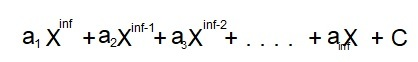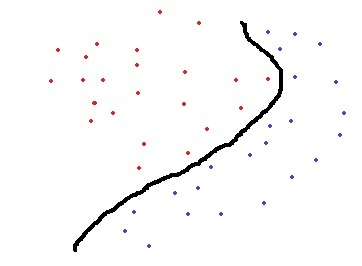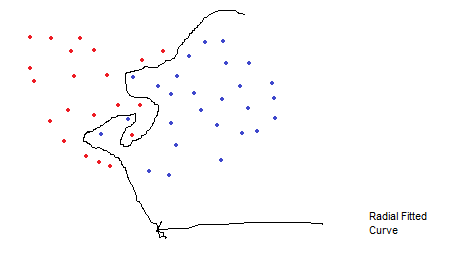# Radial Basis Function Kernel – Machine Learning

Radial Basis Kernel is a kernel function that is used in machine learning to find a non-linear classifier or regression line.

What is Kernel Function?
Kernel Function is used to transform n-dimensional input to m-dimensional input, where m is much higher than n then find the dot product in higher dimensional efficiently. The main idea to use kernel is: A linear classifier or regression curve in higher dimensions becomes a Non-linear classifier or regression curve in lower dimensions.

Mathematical Definition of Radial Basis Kernel:where x, x’ are vector point in any fixed dimensional space.
But if we expand the above exponential expression, It will go upto infinite power of x and x’, as expansion of ex contains infinite terms upto infinite power of x hence it involves terms upto infinite powers in infinite dimension.
If we apply any of the algorithms like perceptron Algorithm or linear regression on this kernel, actually we would be applying our algorithm to new infinite-dimensional datapoint we have created. Hence it will give a hyperplane in infinite dimensions, which will give a very strong non-linear classifier or regression curve after returning to our original dimensions.polynomial of infinite power

So, Although we are applying linear classifier/regression it will give a non-linear classifier or regression line, that will be a polynomial of infinite power. And being a polynomial of infinite power, Radial Basis kernel is a very powerful kernel, which can give a curve fitting any complex dataset.

Why Radial Basis Kernel Is much powerful?
The main motive of the kernel is to do calculations in any d-dimensional space where d > 1, so that we can get a quadratic, cubic or any polynomial equation of large degree for our classification/regression line. Since Radial basis kernel uses exponent and as we know the expansion of e^x gives a polynomial equation of infinite power, so using this kernel, we make our regression/classification line infinitely powerful too.

Some Complex Dataset Fitted Using RBF Kernel easily:References:

My Personal Notes arrow_drop_upCheck out this Author's contributed articles.

If you like GeeksforGeeks and would like to contribute, you can also write an article using contribute.geeksforgeeks.org or mail your article to contribute@geeksforgeeks.org. See your article appearing on the GeeksforGeeks main page and help other Geeks.

Please Improve this article if you find anything incorrect by clicking on the "Improve Article" button below.

Article Tags :
Practice Tags :

Be the First to upvote.

Please write to us at contribute@geeksforgeeks.org to report any issue with the above content.